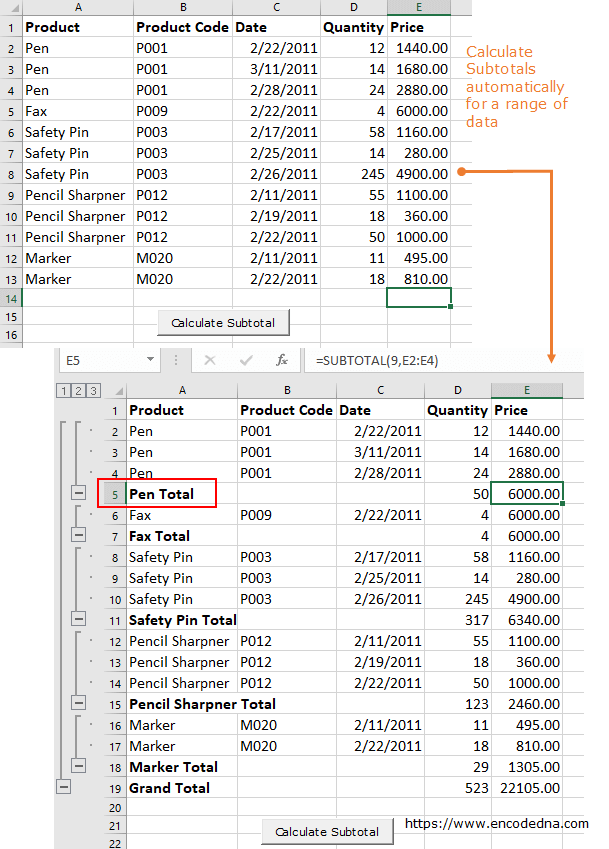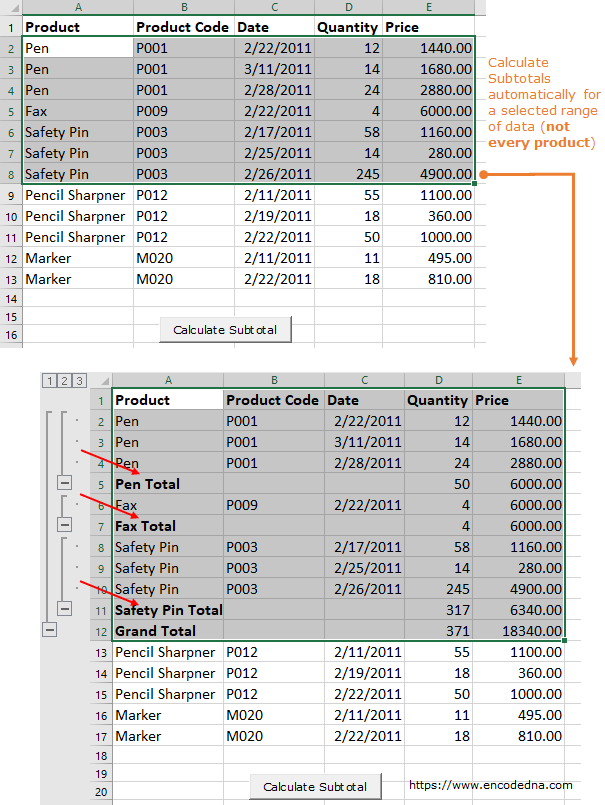﻿ How to calculate the Subtotals for a Range a data in Excel using VBA

# How to calculate the Subtotals for a Range a data in Excel using VBA

In Excel, there is an in-built feature called the Subtotal (under the Data tab) which when used can automatically insert subtotals and grand total for a selected range of data. However, if you are a VBA programmer, you can further automate this process using a simple, one line macro.

The image below explains it.The Macro

Let us assume, I have a range of data with few columns (see the above image again). I want to show the Subtotal of Price for each product and finally at the last row show the grand total, with the click of a button.

Note: The result will be the same like the Subtotal feature that Excel provides. We are doing this using a Macro.

```Option Explicit

Private Sub CommandButton1_Click()
showSubTotal
End Sub

Sub showSubTotal()
Worksheets("sheet1").Activate

Range("A1:E" & Cells(Rows.Count, "E").End(xlUp).Row).Subtotal _
GroupBy:=1, Function:=xlSum, TotalList:=Array(5, 4), _
Replace:=True, PageBreaks:=False

End Sub```

In the above macro, I am using the Range() method where I have defined a range from column A to E. This is fixed, kind of hardcoded. Therefore, every time I click the button, it will show the subtotal and grand total of that particular range, A to E.

Now here’s another scenario. I don’t want to show the subtotal of all the products, only a few. For example, I want to insert subtotal to selected products only, like Pens, Fax and Safety pins.

To do this I can use the same method (that I used in the above example) using the Selection property.

```Option Explicit

Private Sub CommandButton1_Click()
showSubTotal
End Sub

Sub showSubTotal()
Worksheets("sheet1").Activate

Selection.Subtotal _
GroupBy:=1, Function:=xlSum, TotalList:=Array(5, 4), _
Replace:=True, PageBreaks:=False
End Sub```Remember, if you are using the Selection property, you have to first select a range and then click the button (or whatever method you are using). Or else it will throw an error.